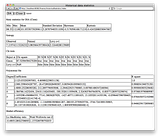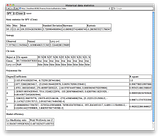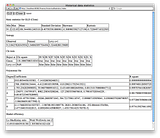# Are stock markets efficient?

This entry is part of 15 in the series Grails Finance

Grails finance 0.9

According to theory a market is efficient, if it has random prices. So tests for market efficiency come down to testing for randomness. Obviously the same goes, if stock prices follow some distribution that is not random.

## Services

So I made some services to calculate statistic parameters

• chiSquareService
• theoDistService
• descriptiveStatisticsService
• loMacKinlayService
• waldWolfowitzService
• entropyService
• polynomialFitService

### Chi Square Service

This service uses the ChiSquareTestImpl of Apache Commons Math to perform goodness of fit test for several distributions. My goodness!

```1 2 3 4 5 6 7 8 9 10 11 12 13 14 15 16 17 18 19 20 21 22 23 24 25 26 27 28 29 30 31 32 33 34 35 36 37 38 39 40 ... def chiSquare(expected, observed) { return instance.chiSquare(expected as double[], observed as long[]) }   def canBeRejected(expected, observed) { def rejections = [] expected = expected as double[] observed = observed as long[] rejections &lt;&lt; instance.chiSquareTest(expected, observed, 0.01) rejections &lt;&lt; instance.chiSquareTest(expected, observed, 0.02) rejections &lt;&lt; instance.chiSquareTest(expected, observed, 0.03) rejections &lt;&lt; instance.chiSquareTest(expected, observed, 0.04) rejections &lt;&lt; instance.chiSquareTest(expected, observed, 0.05) rejections &lt;&lt; instance.chiSquareTest(expected, observed, 0.06) rejections &lt;&lt; instance.chiSquareTest(expected, observed, 0.07) rejections &lt;&lt; instance.chiSquareTest(expected, observed, 0.08) rejections &lt;&lt; instance.chiSquareTest(expected, observed, 0.09)   return rejections } ... void testChiSquare() { def expected = [2, 1]as double[] def observed = [2, 1]as long[] assertEquals 0, chiSquareService.chiSquare(expected, observed)   expected = [2/3.0, 1/3.0]as double[] observed = [2, 1]as long[] assertEquals 0, chiSquareService.chiSquare(expected, observed), 0.001 }   void testCanBeRejected() { def expected = [2, 1]as double[] def observed = [2, 1]as long[] def rejections = chiSquareService.canBeRejected(expected, observed)   assertFalse rejections } ...```

### Theoretical distribution service

This service calculates pdf functions for the Levy and Gauss distributions. Apache Commons Math already has a NormalDistributionImpl, so I am using that.

```1 2 3 4 5 6 7 8 9 10 11 12 13 14 15 16 17 18 19 20 21 22 23 24 25 26 27 28 29 30 31 32 33 34 35 36 37 38 39 40 41 42 43 44 45 46 ... def normalPDF(mean, stddev, x) { return new NormalDistributionImpl(mean, stddev).density(x) }   def gaussExpected(mean, stddev, xpoints) { def expected = [] def sortedPoints = xpoints.sort()   sortedPoints.each { expected &lt;&lt; normalPDF(mean, stddev, it) }   return expected }   def levyExpected( c, xpoints , mu) { def expected = [] def sortedPoints = xpoints.sort()   sortedPoints.each { expected &lt;&lt; levyPDF( c, it, mu ) }   return expected }   def levyPDF( c, x, mu ) { def constant = c / (2 * Math.PI) def exponent = Math.exp(-1 * c / (2 * (x - mu) )) def pow32 = Math.pow(x - mu, 3/2) return Math.sqrt( constant ) * exponent / pow32 } ... void testNormalPDF() { assertEquals 1/ Math.sqrt(2 * Math.PI), theoDistService.normalPDF(0, 1, 0) assertEquals 1/ Math.sqrt(2 * Math.PI), theoDistService.normalPDF(1, 1, 1) }   void testGaussExpected() { def xpoints = [1 : 0, 0 : 0].keySet() def result = theoDistService.gaussExpected(0, 1, xpoints) assertEquals result, 1/ Math.sqrt(2 * Math.PI) assertEquals result, Math.exp(-0.5)/ Math.sqrt(2 * Math.PI) } ...```

### Descriptive statistics service

The descriptive statistics service calculate basic statistics parameters such as mean, standard deviation etc. I use the DescriptiveStatistics class for that. For some reason you have to add values to it one by one.

```1 2 3 4 5 6 7 8 9 10 11 12 13 14 15 16 17 18 19 ... def addValues(doublesList) { stats = new DescriptiveStatistics() doublesList.each { stats.addValue(it) } }   def stats(doubles) { def map = [:] addValues(doubles) map["mean"] = stats.getMean() map["stddev"] = stats.getStandardDeviation() map["kurtosis"] = stats.getKurtosis() map["skewness"] = stats.getSkewness() map["max"] = stats.getMax() map["min"] = stats.getMin()   return map } ...```

### Lo MacKinlay Service

The Lo MacKinlay variance ratio uses as base parameter the delta of the price logarithm. For instance, the logarithm of the closing price of today minus the logarithm of the closing price of yesterday. We make two data sets – one with all the data and another with any odd item from the original data removed. The theory says that the variance ratio of these sets with 1 subtracted from it shold be equal to 0, for random data.

```1 2 3 4 5 6 7 8 9 10 11 12 13 14 15 16 17 18 19 20 21 22 23 24 25 26 27 28 29 30 31 32 33 34 35 36 37 38 39 40 41 42 43 44 45 46 47 48 49 50 51 52 53 54 55 56 57 58 59 60 61 ... def evenItems(input) { def evenItems = []   for( int i in (0..&lt;input.size() ).step(2) ) evenItems &lt;&lt; input[i]   return evenItems }   def lnService   double variance(input) { def deltas = lnService.deltaLn(input) return StatUtils.variance(deltas as double[]) }   def ratio(input) { def doubles = input as double[] def totalVariance = variance( doubles ) def evenVariance = variance( evenItems(doubles) )   return totalVariance / evenVariance - 1 } ... void testEvenItems() { def items = 0..4 def outcome = loMacKinlayService.evenItems(items)   assertEquals "Incorrect size", 3, outcome.size() assertEquals outcome, 0 assertEquals outcome, 2 assertEquals outcome, 4 }   void testVariance() { def input = [ Math.exp(1), Math.exp(2), Math.exp(3) ] def mu = 1 def sigma2 = Math.pow( 2 - 1 - mu, 2) sigma2 += Math.pow( 3 - 2 - mu, 2) sigma2 /= 2   def outcome = loMacKinlayService.variance(input) assertEquals sigma2, outcome, 0.001 }   void testRatio() { def uniform = [] def rdi = new RandomDataImpl()   for( i in 0..1000 * 1000 ) uniform &lt;&lt; rdi.nextUniform(1, 100)   def uniformRatio = loMacKinlayService.ratio( uniform ) assertEquals 0, uniformRatio, 0.01 } ...```

### Wald Wolfowitz service

The Wald Wolfowitz runs test looks at sequences like +++—+++. – can be a negative price change and + a positive one. Actually this is a bit arbitrary, you could also define + to be a value larger than the median and – a value smaller than the median. However, if you have no change or the value is equal to the median than you are violating these constraints. In a large sample, hopefully this situation does not occur too frequently. It is easy to calculate the mean and deviation for the number of runs of a random distribution. From this the Z score follows, that allows us to accept or reject randomness.

```1 2 3 4 5 6 7 8 9 10 11 12 13 14 15 16 17 18 19 20 21 22 23 24 25 26 27 28 29 30 31 32 33 34 35 36 37 38 39 40 41 42 43 44 45 46 47 48 49 50 51 52 53 54 55 56 57 58 59 60 61 62 63 64 65 66 67 68 69 70 71 72 73 74 75 76 77 78 79 80 81 82 83 84 85 86 87 88 89 90 91 92 93 94 95 96 97 98 99 100 101 102 103 104 105 106 107 108 109 110 ... def totalRuns(changes) { def runs = 1 def posOrNeg = sign( changes )   for( i in 1..&lt;changes.size()) { def currSign = sign( changes[i] )   if(currSign != posOrNeg &amp;&amp; currSign != 0) runs++   posOrNeg = currSign }   return runs }   def sign(val) { def posNegOr0 = 0   if(val &lt; 0) posNegOr0 = -1 if(val &gt; 0) posNegOr0 = 1   return posNegOr0 }   def neg(changes) { def negList = changes.findAll { it &lt; 0 } return negList.size() }   def pos(changes) { def posList = changes.findAll { it &gt; 0 } return posList.size() }   def Z(list) { def deltas = subtractService.minPrev(list) def minuses = neg(deltas) def pluses = pos(deltas) def runs = totalRuns(deltas) def expected = expectedRuns( minuses, pluses) def deviation = stddev(minuses,pluses)   return (runs - expected) / deviation }   def expectedRuns(n1, n2) { return 2 * n1 * n2 / (n1 + n2) + 1 }   def stddev(n1, n2) { def product = n1 * n2 def up = 2 * product * (2 * product - n1 - n2)   def sum = n1 + n2 def down = sum * sum * ( sum - 1)   return Math.sqrt( up / down ) } ... def list = [1,-1,-1,-1,1,1,1,0,1,1,-1,-1,-1,1,0,-1,1,-1,0,1]   void testRuns() { def sublist = [1, -1, -1, -1] def runs = waldWolfowitzService.totalRuns(sublist) assertEquals 2, runs   sublist = [1, -1, -1, -1, 1, 1, 1, 0, 1, 1] runs = waldWolfowitzService.totalRuns(sublist) assertEquals 4, runs   runs = waldWolfowitzService.totalRuns(list) assertEquals 10, runs }   void testNeg() { def minuses = waldWolfowitzService.neg(list) assertEquals 8, minuses }   void testPos() { def pluses = waldWolfowitzService.pos(list) assertEquals 9, pluses }   void testZ() { def uniform = [] def rdi = new Random()   for( i in 0..255) uniform &lt;&lt; rdi.nextDouble()   def z = waldWolfowitzService.Z(uniform) println z assertTrue z &lt; 1.645 }   void testExpectedRuns() { def runs = waldWolfowitzService.expectedRuns(8, 9) assertEquals 9.47, runs, 0.01 }   void testStddev() { def sigma = waldWolfowitzService.stddev(8, 9) assertEquals 1.99, sigma, 0.01 } ...```

### Entropy service

We can define a so called information entropy. Entropy is a measure of disorder or randomness. The higher the entropy, the higher the randomness. You can find the entropy formulas for several stable distributions on Wikipedia.

```1 2 3 4 5 6 7 8 9 10 11 12 13 14 15 16 17 18 19 20 21 ... def normalEntropy(sigma) { return Math.log( 2 * Math.PI * Math.E * sigma * sigma )/ 2 }   def entropy(p) { def s = 0   p.each { if( it &gt; 0) { s += (it / p.size() ) * Math.log(it / p.size()) } }   return -1 * s }   def levyEntropy( c ) { return (1 + 3 * Gamma.GAMMA + Math.log( 16 * Math.PI * c * c )) / 2 } ...```

### Polynomial fit service

The polynomial fit service fits the data to a polynomial of a certain degree and calculates the R square for the fit.

```1 2 3 4 5 6 7 8 9 10 11 12 13 14 15 16 17 ... def fit(degree, vals) { PolynomialFitter fitter = new PolynomialFitter(degree, new LevenbergMarquardtOptimizer())   for(i in 0 ..&lt;vals.size() ) fitter.addObservedPoint( i + 1, vals[i], i + 1)   PolynomialFunction fitted = fitter.fit()   def regression = new SimpleRegression()   for(i in 0 ..&lt;vals.size() ) regression.addData(vals[i], fitted.value(vals[i]))   return [coefficients : fitted.getCoefficients(), rsquare: regression.getRSquare()] } ...```

## Controller

The controller collects the data of the services and provides on request from the view a map with all the necessary information.

```1 2 3 4 5 6 7 8 9 10 11 12 13 14 15 16 17 18 19 20 21 22 23 24 25 26 27 28 29 30 31 32 33 34 35 36 37 38 39 40 41 42 43 44 45 46 47 48 49 50 51 52 53 54 55 56 57 58 59 60 61 62 63 64 65 66 67 ... def calcFrequence(vals, fieldVar) { def frequence = frequenceService.frequence(vals)   return frequence }   def doubleValues(map) { return map.values() as double[] }   def doubleKeys(map) { def keys = map.keySet() def doubleKeys = [] keys.each { doubleKeys &lt;&lt; Double.valueOf(it) }   return doubleKeys }   def makeResponse(vals) { def frequence = calcFrequence(vals, params.fieldVar) def statsMap = descriptiveStatisticsService.stats(vals) def normalExpected = theoDistService.gaussExpected(statsMap["mean"], statsMap["stddev"], doubleKeys(frequence))   def chi2 = chiSquareService.chiSquare(normalExpected, frequence.values()) def rejections = chiSquareService.canBeRejected(normalExpected, frequence.values())   def lomac = loMacKinlayService.ratio(vals) def waldWolf = waldWolfowitzService.Z(vals as double[])   def sampleEntropy = entropyService.entropy(frequence.values()) def naturalEntropy = entropyService.normalEntropy(statsMap["stddev"])   def levyConstant = 1 def levyEntropy = entropyService.levyEntropy( levyConstant ) def levyExpected = theoDistService.levyExpected( levyConstant, doubleKeys(frequence), statsMap["mean"])   def levyRejections = chiSquareService.canBeRejected( levyExpected, frequence.values()) def levyChi2 = chiSquareService.chiSquare(levyExpected, frequence.values())   def fits = []   for(degree in 1..6) fits &lt;&lt; polynomialFitService.fit(degree, vals)   return [chiSquare : chi2, statsMap : statsMap, rejections : rejections, lomac : lomac, waldWolf : waldWolf, sampleEntropy : sampleEntropy, naturalEntropy: naturalEntropy, levyEntropy : levyEntropy, levyChi2 : levyChi2, levyRejections : levyRejections, fits : fits] }   def index = { if(params.fieldVar != null) { def vals = historicalQueryService.queryVals( params.select1, params.fieldVar); return makeResponse(vals) } } ...```

## The view

```1 ...```

Basic statistics for \${params.select1} (\${params.fieldVar})

 Min Max Mean Standard Deviation Skewness Kurtosis \${statsMap[“min”]} \${statsMap[“max”]} \${statsMap[“mean”]} \${statsMap[“stddev”]} \${statsMap[“skewness”]} \${statsMap[“kurtosis”]}

Entropy

 Observed Natural Levy c=1 \${sampleEntropy} \${naturalEntropy} \${levyEntropy}

Chi tests

 Reject at Chi square \${100 – it} % Gauss \${chiSquare} \${rejections[it]} Levy c=1 \${levyChi2} \${levyRejections[it]}

Polynomial fits

 Degree Coefficients R square \${it + 1} \${fits[it][“coefficients”].toString()} \${fits[it][“rsquare”].toString()}

Market efficiency

 Lo MacKinlay ratio Wald Wolfowitz test Z \${lomac} \${waldWolf}
```1 ...```

## Result

Here are the statistics of the closing prices of DIA, SPY and GLD.## Conclusions

The main conclusion is that this is all so not scientific ;). There is just not enough data. So are markets random/efficient? Sometimes, it depends. I don’t know! By the way I am pretty sure that I made mistakes in the calculations. I apologize for that, but you know I have been busy. If you find bugs, please let me know!